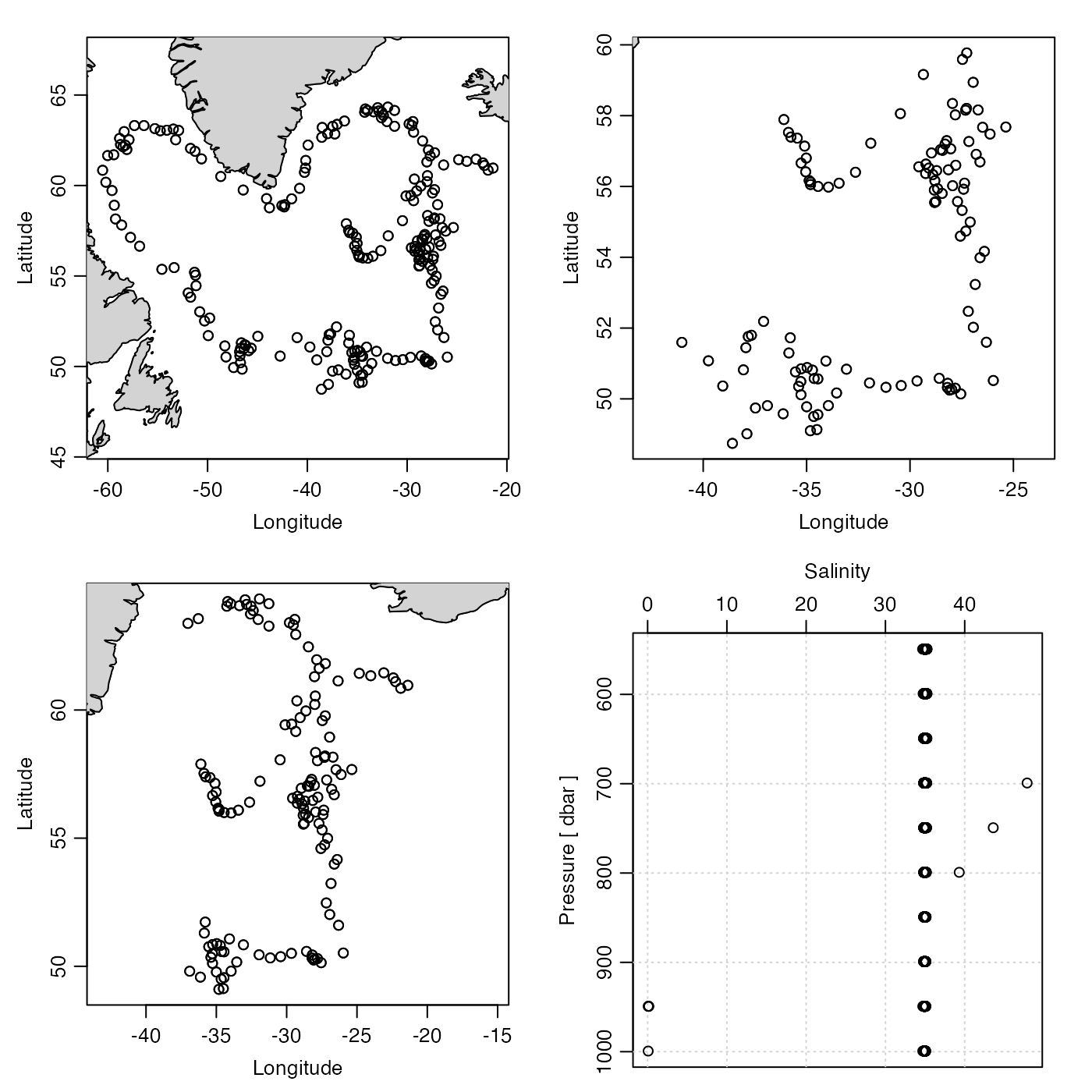Subset an argo object, either by selecting just the "adjusted" data or by subsetting by pressure or other variables.

# S4 method for argo
subset(x, subset, ...)

## Arguments

x

an argo object.

subset

An expression indicating how to subset x.

...

optional arguments, of which only the first is examined. The only possibility is within, a polygon enclosing data to be retained. This must be either a list or data frame, containing items named either x and y or longitude and latitude; see Example 4. If within is given, then subset is ignored.

An argo object.

## Details

If subset is the string "adjusted", then subset replaces the station variables with their adjusted counterparts. In the argo notation, e.g. PSAL is replaced with PSAL_ADJUSTED; in the present notation, this means that salinity in the data slot is replaced with salinityAdjusted, and the latter is deleted. Similar replacements are also done with the flags stored in the metadata slot.

If subset is an expression, then the action is somewhat similar to other subset functions, but with the restriction that only one independent variable may be used in in any call to the function, so that repeated calls will be necessary to subset based on more than one independent variable. Subsetting may be done by anything stored in the data, e.g. time, latitude, longitude, profile, dataMode, or pressure or by profile (a made-up variable), id (from the metadata slot) or ID (a synonym for id). Note that subsetting by pressure preserves matrix shape, by setting discarded values to NA, as opposed to dropping data (as is the case with time, for example).

## Sample of Usage


# Example 2: restrict attention to delayed-mode profiles.
par(mfrow=c(1, 1))
plot(subset(argo, dataMode == "D"))

par(mfrow=c(1, 2))
plotTS(argo)

# Example 2. Subset by a polygon determined with locator()
par(mfrow=c(1, 2))
plot(argo, which="map")
# Can get a boundary with e.g. locator(4)
boundary <- list(x=c(-65, -40, -40, -65), y=c(65, 65, 45, 45))
argoSubset <- subset(argo, within=boundary)
plot(argoSubset, which="map")

Other things related to argo data: [[,argo-method, [[<-,argo-method, argo-class, argoGrid(), argoNames2oceNames(), argo, as.argo(), handleFlags,argo-method, plot,argo-method, read.argo.copernicus(), read.argo(), summary,argo-method

Other functions that subset oce objects: subset,adp-method, subset,adv-method, subset,amsr-method, subset,cm-method, subset,coastline-method, subset,ctd-method, subset,echosounder-method, subset,lobo-method, subset,met-method, subset,oce-method, subset,odf-method, subset,rsk-method, subset,sealevel-method, subset,section-method, subset,topo-method, subset,xbt-method

Dan Kelley

## Examples

library(oce)
data(argo)

# Example 1: subset by time, longitude, and pressure
par(mfrow = c(2, 2))
plot(argo)
plot(subset(argo, time > mean(time)))
plot(subset(argo, longitude > mean(longitude)))
plot(subset(argoGrid(argo), pressure > 500 & pressure < 1000), which = 5)